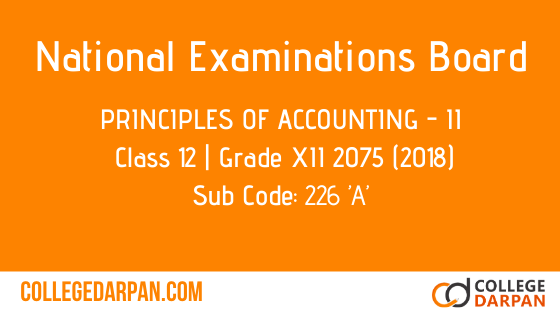# NEB- Grade XII 2075 (2018) Principles of Accounting – II (226 ’A’)

Subject Code: 226 ’A’

2075 (2018)

Principles of Accounting – II

Candidates are required to give their answers in their own words as far as practicable. The figures in the margin indicate full marks.

Time – 3 hrs

Full Marks – 100

Pass Marks – 35 (Only for partial students)

(Group ‘A’)

( Attempt  all the questions)

1. (Define prospectus and mentions any two contents to be stated in prospectus.)  [1+2=3]

2. (Give the meaning of Debenture.)   

3. (State the parties interested in Financial statement.)   

4. (Write the meaning of Radio Analysis.)  

5. (Briefly, explain any three objectives of Cost Accounting.)   

6. (With suitable example, write the meaning of fixed cost.) 

7. (Define time rate system of wages payment. How is it determined?   

8. (Give the meaning of apportionment of overhead.) 

9. (A company forfeited 80 shares of Rs. 10 each, issued at 10% discount, for non-payment of final call of Rs. 3 per share. Out of these shares 60 shares were re-issued at Rs. 8 per share as fully paid.)

(Required):   [1+1+1=3]

(Entries for forfeiture, re-issue and transfer of share)

10. A company issued 4000 shares of Rs. 100 each at a premium of Rs. 10 per share payable Rs. 20 on application, Rs. 50 on allotment (including premium) and Rs. 40 on final call per share.

Application were received for 6000 shares. These shares were alloted on prorata basis to the applicants for 5000 shares and remaining were rejected. Excess application money were untilized towards on allotment. All money were duly received.

(Required): [2+2+2=6]

(Entries for application, allotment and final call)

11. P Company Ltd. issued 5000 shares of 100 each at a premium of 20% to purchase the following assets and liabilities of Q company ltd.

(Fixed Assets):        Rs. 600,000

(Loan):                               Rs. 100,000

(Current Assets):      Rs. 200,000

(Creditors):                      Rs. 50,000

(Required):  [2+1=3]

(Entries for purchase of assets and liabilities.)

12. A Company Ltd. issued 4000, 10% debentures of Rs. 100 each at par. These debentures were redeemed after 7 years at 10% premium.

(Required):  [2+2=4]

(Entries for issue and redemption of debentures)

13. The Trial Balance of a company is given below:

a) Commission earned but not received: Rs. 3,000

b) Prepaid rent Rs. 6,000 was expired.

(Required):  [2+6=8]

ii) (Work Sheet)

14. Trial Balance of Ganesh Company Ltd. as on 31st Dec. 2017 is given below:

a) (Depreciation on Furniture): by 10%

b) (Outstanding wages): Rs. 2,000

c) (Closing stock): Rs. 18,000

d) (Proposed dividend): 10%

e) (Create a provision for bad debts 5%)

(Required):  [3+4+1+4=12]

ii) (Profit and Loss Account)

iii) (Profit and loss Appropriation Account)

iv) (Balance Sheet)

15. Following information are provided:

(Stock): Rs. 200,000

(Sales):  Rs. 700,000

(Net Profit):  Rs. 100,000

(Creditors):  Rs. 125,000

(Cash):  Rs. 50,000

(Debtors):  Rs. 150,000

(Inventory Turnover Ratio): 5 times

(Fixed Assets turnover): 2.5 times

(Required):  [1+1+1+2=5]

i) (Current Ratio)

ii) (Liquid Ration)

iii) (Debtors Turnover Ratio)

iv) (Fixed Assets)

16. Following information are given:

a) (Dividend paid 2nd year): Rs. 25, 000

b) (Depreciation charge): Rs. 15, 000

c) (Fixed assets purchase during 2nd year): Rs. 70, 000

(Required):  [3+2=5]

i) (Funds from operation)

ii) (Funds flow statement)

17. The Balance Sheet of a company for two years are as under:

a) (Sales Revenue): Rs. 13,00,000

b)(Cost of goods sold):  Rs. 700,000

d) (Purchased of fixed assets):  Rs. 300,000

(Required):  [5+2+2+1=10]

(Cash flow statement)

18. The following transactions were taken from the store for the month of poush:

(Poush 1): (Opening balance): 400 units for Rs. 2000

(Poush 3): (Purchase): 600 units @ Rs. 6 each

(Poush 6): (Issued): 800 units

(Poush 15): (Return from department): 70 units

(Poush 22): (Purchase): 800 units @ Rs. 7 each

(Poush 25): (Issued): 830 units

(Poush 28): (Store verification surplus): 10 units

(Required):  

Store ledger under First In First Out (FIFO) Method.

19. The following information are given:

(Annual requirement): 36,000 units

(Ordering cost per order): Rs. 60

(Cost per unit): Rs. 100

Carrying cost per unit 10% of inventory cost

(Required):  

(Economic Order Quantity)

20. Standard output in a day of 8 hours is 56 units. Rate per unit is Rs. 100 and a worker worked 180 hours in a month.

(Required):  

(Monthly wages of a worker)

21. The details of manufacturing and other costs are as follow:

(Direct material): Rs. 1,00,000

Direct labour): Rs. 80,000

Following estimate costs were made for submitting tender:

(Direct material): Rs. 60,000

(Direct labour): Rs. 40,000

Factory overhead based on direct labour and other overhead based on works cost and factory wants earn 25% profit on sales.

(Required):  [3+7=10]

i) (Cost sheet)

ii) (Tender sheet)

22. Net profit as per financial account was Rs. 60,000. On reconciliation, the following facts were noticed.
a) Factory overhead under recorded in cost account Rs. 8,000.

b) Interest expense recorded in financial account Rs. 3,000.

c) Over valuation of opening stock in financial account Rs. 6,000.

d) Income tax paid Rs. 8,500).

(Required):  

(Reconciliation between cost and financial account)

Download Questions only PDF: NEB- GRADE XII 2075 (2018) PRINCIPLES OF ACCOUNTING – II (226 ’A’): PRINCIPLES OF ACCOUNTING – II (226 ’A’) Question only.